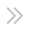InsightsEducationTrading Fundamentals
Minutes
Invalid Date Invalid Date## Definition of a Pip

The unit of measurement to express the change in value between two currencies is called a “pip”, also referred to as price interest point. A pip is the smallest whole unit price move that an exchange rate can make, based on forex market convention.

Most currency pairs are priced out to four decimal places and a single pip is in the last (fourth or second) decimal place.

There are also brokers that quote currency pairs beyond the standard “4 and 2” decimal places to “5 and 3” decimal places, like Mitrade. These are referred to as fractional pips, also called “points” or “pipettes.”fig 1. For currency pairs and quotes displayed to 5 decimal places

Suppose the price of EUR/USD was 1.13452. After a price movement, if the price of EUR/USD is now 1.13482, it changes by 0.00030, 30 points or 3 pips (from 1.13452 to 1.13482).fig 2. For currency pairs and quotes displayed to 3 decimal places

There are also currency pairings with 3 decimal places such as USD/JPY. Suppose the price of USD/JPY was 143.118 and after a price movement, if the price of USD/JPY rose to 143.178, it changed by 0.60, 60 points or 6 pips (from 143.118 to 143.178).

## The calculation of the value of a pip depends on the three elements:

1. Currency Pairing / Instrument
3. Currency Price

Any minute changes on the the above mentioned factors can cause a change in the calculation on the value of a pip and the trade.

A trader opened a long position (buy trade) of 50,000 dollars on the pair USD/CAD at price 1.3050. Subsequently the price risen, the trader closed his trades and profited 50pips. Assuming the traders’ base currency is denominated in USD, here are the steps to calculate the profit/loss.

(1) Determine the value of one pip based on quote price (CAD | Canadian Dollar):

Volume of Trade x 1 pip = Dollar Value (Quote) / pip

(2) Calculate the value one pip by using base currency (USD | US Dollar):

Value 1 pip based on quote price (CAD) / Currency Price = Pip Value / USD
5/1.3050 = 3.83 USD/pip

(3) Determine the total profit and loss of the trade:

Number of pips in profit and loss x Pip Value in USD = Trade Profit and Loss in USD
50 x 3.83 = 191.50 USD

Example 2 : USD/JPY (US Dollar against Japanese Yen)

A trader opened a long position (buy trade) of 50,000 dollars on the pair USD/JPY at price 123.456. Subsequently the price dropped to 123.256, the trader closed his trades and loss 20pips. Assuming the traders’ base currency is denominated in USD, here are the steps to calculate the profit/loss.

(1) Determine the value of one pip based on quote price (JPY | Japanese Yen):

50,000 x 0.01 = 500yen / pip

(2) Calculate the value one pip by using base currency (USD | US Dollar):

500 / 123.256 = 4.057 US dollars/pip

(3) Determine the total profit and loss of the trade:

-20pip loss x 4.057USD/pip = -81.14USD

As a result, the trader lost \$ 81.14.

* The content presented above, whether from a third party or not, is considered as general advice only.  This article should not be construed as containing investment advice, investment recommendations, an offer of or solicitation for any transactions in financial instruments.

Table of Content